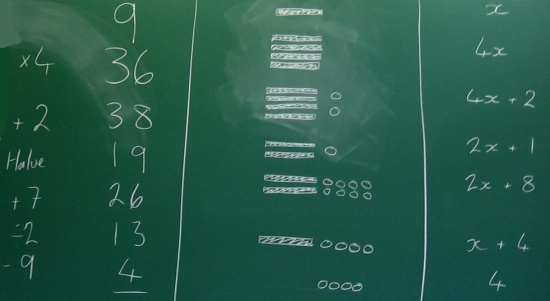#### You may also like### Days and Dates

Investigate how you can work out what day of the week your birthday will be on next year, and the year after...### Summing Consecutive Numbers

15 = 7 + 8 and 10 = 1 + 2 + 3 + 4. Can you say which numbers can be expressed as the sum of two or more consecutive integers?### Always the Same

Arrange the numbers 1 to 16 into a 4 by 4 array. Choose a number. Cross out the numbers on the same row and column. Repeat this process. Add up you four numbers. Why do they always add up to 34?

### Why do this problem?

This problem provides a great way of introducing a very useful way of representing operations on unknown numbers visually and symbolically (algebraically). The interactivity provides a hook to engage students' curiosity, and the visual representation that appears on the right hand side of the interactivity offers a way of explaining what is going on in order to satisfy that curiosity.

### Possible approach

Ask learners to each think of a number and read out the following instructions:
Think of a number
Double
Halve
Take away the number you started with
What did you end up with?

Look surprised, when all of them reveal they have ended up with the same number.
"Perhaps none of you started with a fraction, or decimal, or a negative number - shall we try it again with one of those?"
More surprise that they still end up with the same number.

Now show the interactivity, or alternatively draw the representation that appears on the right hand side in the interactivity, and ask learners to think about how the visual representation helps us to understand what is happening. Give them a couple of minutes to discuss in pairs, and then draw the class together to share their insights.

Next, suggest that the visual representation might be too time-consuming, particularly if we'd added and multiplied by larger numbers. Introduce a parallel representation using algebra:
$$x$$ $$x+3$$ $$2(x+3) \text{ or }2x+6$$ $$2x+10$$ $$x+5$$ $$5$$

Now invite three members of the class up to the board to each use a different representation simultaneously for some other "think of a number" sets of instructions.
For example:
Think of a number
Multiply by 4
Halve it
Divide it by 2
Take away the number you first thought of.

This is what the board might look like when the three learners have finished:Finally, challenge the class to come up with their own examples of "Think of a number" instructions, which will lead to an anticipated solution. Encourage them to record their working using both the visual and the symbolic representation.

At the end of the lesson, a selection of students can read out their instructions for the class to try, and then reveal the anticipated solution (written in advance) with a flourish.

### Key questions

How can I use the visual or symbolic representation to explain the "Think of a number" puzzle?
How can I use the visual or symbolic representation to create a "Think of a number" puzzle?

### Possible extension

Your Number Was... is a suitable follow-up activity.
Think of Two Numbers is a challenging extension.

### Possible support

Spend time working with the visual representations before introducing symbolic notation.